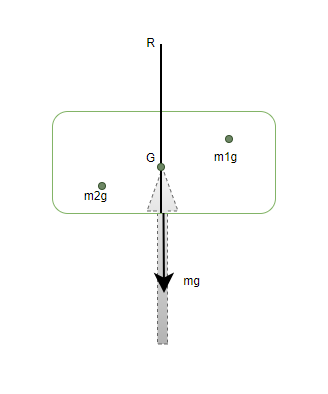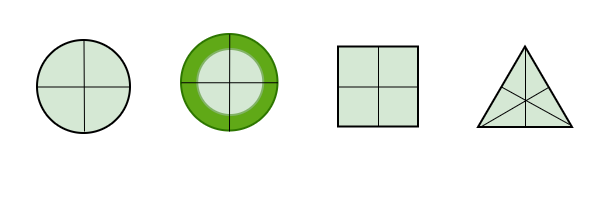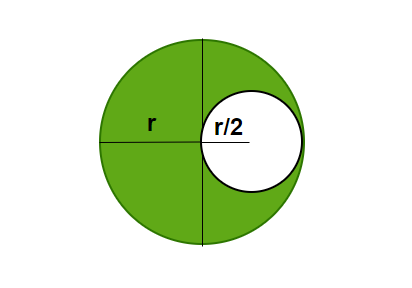Open in App
Not now

# Centre of Gravity

• Last Updated : 06 Oct, 2021

Often while dealing with mechanics and gravity, engineers and scientists come across solid bodies which do not point masses. Such bodies are bigger in size and cannot be considered as point masses in the calculations. In such cases, it is assumed there is a certain point where all the mass of the body is concentrated and gravity also acts on a certain point. This assumption allows us to simplify complex calculations. These points are called the center of mass and the center of gravity respectively. Let us look at these concepts in detail.

### Centre of Gravity

Theoretically, the center of gravity is considered to be a point where the weight of the body acts. Finding this point is very essential in bodies that have large sizes and often non-uniform mass distribution. This point allows us to predict the motion of such bodies under the influence of gravity. If a body is balanced by this point, it is rotational and translational equilibrium. Consider the example of the body given in the figure below,The figure shows cardboard being balanced by a pencil kept below it. The tip of the pencil applies a reaction force on the point which keeps the cardboard in translational equilibrium. It is also in rotational equilibrium, it can be verified by the fact that the object does not have any angular acceleration. Let us assume that the cardboard is made up of very small individual point masses m1, m2, m3…

Gravity is acting on all these point masses creating torque around the point. The Center of Gravity (CG) is located such that the total torque on CG due to forces on individual point masses is zero. If ri is the position vector of the ith particle with respect to the CG, then the torque on it due to the gravitational force is given by,For a point that is center of gravity, the total torque about that point must be zero,Notice that in the equation above, the gravitational force “g” is the same for every point. It can come out of the equation as a common factor to all the terms.Since g is a constant and non-zero. It can be concluded that,### Center of Mass and Center of Gravity

Its position is defined relative to an object or the system of objects whose Centre of mass is to be calculated. Usually for uniform shapes, it’s their centroid. Let’s start with simple shapes and see where their centers of masses are located. Consider the shapes given in the figure below. It’s easy to guess the Centre of masses for the following shapes. For most of them, the Centre of mass is at their centroid.Notice, for a ring, its Centre of mass lies inside the ring, which means it is necessary that the Centre of mass of a body lies in the body itself.  Now, it is clear that bodies that are uniform and symmetrical have their Centre of masses at their centroid. But for bodies that are not symmetrical and uniform, the answer is not that simple. The Centre of mass for such bodies can be anywhere. To work out the Centre of mass of a complex object. A weighted average of the locations of each mass of the body is taken.

Let’s say there is a body consisting of a set of masses “mi“, each at position ri relative to the location of the Centre of mass.

⇒ rcm =Notice that in case the gravitational force is constant, the position of the center of mass and center of gravity are the same. If a body is so extended that the value of the gravitational force acting on its different parts is different. Then the common factor “g” cannot be taken out and the position of the center of mass is different than the center of gravity.

### Sample Problems

Question 1: Two point masses, m1 = 5Kg and m2 = 2Kg, are located at x = 2 m and x = 6 m respectively. Find the Centre of gravity.

Solution:

The formula for the Centre of gravity is equal to centre of mass when gravity is constant

xcm =m1 = 5Kg,  m2 = 2Kg and x = 2 m and x = 6 m.

M = m1 + m2

⇒ M = 5 + 2 = 7

xcm =⇒ xcm =⇒ xcm =⇒ xcm =Question 2: Twopoint masses, m1 = 5Kg and m2 = 2Kg, are located at y = 10m and y = -5 m respectively. Find the Centre of gravity.

Solution:

The formula for the Centre of gravity is given by,

ycm =m1 = 5Kg,  m2 = 2Kg and y = 10m and y = -5 m.

M = m1 + m2

⇒ M = 5 + 2 = 7

ycm =⇒ ycm =⇒ ycm =⇒ ycm =Question 3: Twopoint masses, m1 = 1Kg and m2 = 2Kg, are located at vector a = 6i + 4j and vector b = -5i + 2j respectively. Find the Centre of gravity.

Solution:

The formula for the Centre of gravity in the vector notation is given by,

rcm =m1 = 1Kg,  m2 = 2Kg and a = 6i + 4j, b = -5i + 2j

M = m1 + m2

⇒ M = 1 + 2 = 3

rcm =⇒ rcm =⇒ rcm =⇒ rcm =⇒ rcm =Question 4: Twopoint masses, m1 = 4Kg and m2 = 2Kg, are located at vector a = i + j and vector b = -i + j respectively. Find the Centre of gravity.

Solution:

The formula for the Centre of gravity in the vector notation is given by,

rcm =m1 = 4Kg,  m2 = 2Kg and a = i + j, b = -i + j

M = m1 + m2

⇒ M = 4 + 2 = 6

rcm =⇒ rcm =⇒ rcm =⇒ rcm =⇒ rcm =Question 5: A disk of radius R/2 is removed from a bigger disk of mass M of radius R. Find the Centre of gravity.Solution:

Since the density of the disk is uniform, the weight is uniformly distributed over all the area.

Mass “m” of the removed disk =Let the distance of the center of mass of the remaining portion be “x”.Question 6: A disk of radius R/4 is removed from a bigger disk of mass M of radius R, in the same manner as the previous figure. Find the Centre of gravity.

Solution:

Since the density of the disk is uniform, the weight is uniformly distributed over all the area.

Mass “m” of the removed disk =Let the distance of the center of gravity of the remaining portion be “x”.My Personal Notes arrow_drop_up